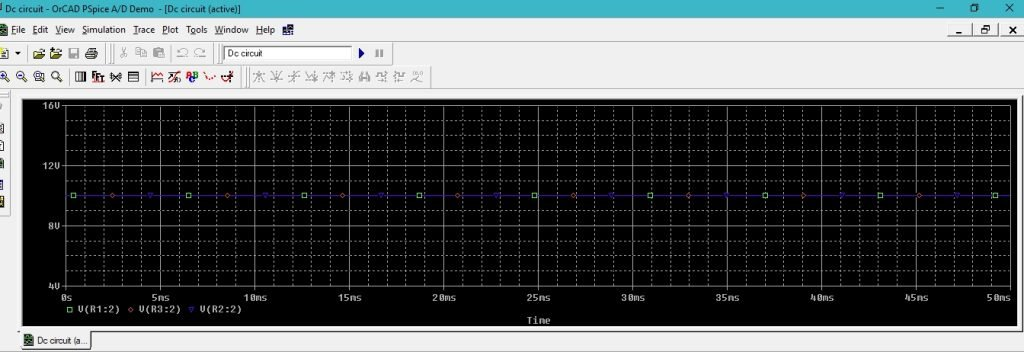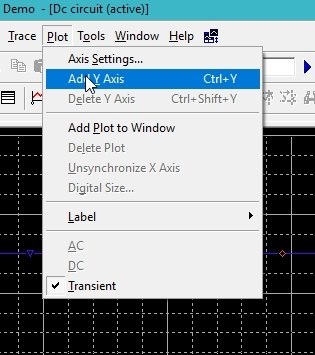# DC circuits analysis with PSpice: tutorial 5

In this tutorial, I will help you analyze a DC circuit. A DC circuit is the one in which the source of the circuit is of direct current rather than alternative current. At the start of the tutorial a brief introduction of DC (direct current) is provided after that a simple DC circuit is implemented in PSPICE and the results were analyzed precisely. At the end of the tutorial you are provided with an exercise to do it by yourself, and in the next tutorials I will assume that you have done those exercises and I will not explain the concept regarding them.

### Introduction to a DC circuits

DC circuit is the circuit in which direct current source is used. Direct current is defined as the constant flow of electric charge traveling from high potential to low potential. Similarly, the circuit that contained electric current flow in only one direction is known as direct current circuit. The relationship between voltage, current and resistance is defined using ohms’ law. Ohms’ law states as

The voltage is directly proportional to the current in a circuit provided that the resistance (and the temperature) remains constant. In this tutorial this law is applied and verified using PSPICE as you will see shortly.

### DC circuits analysis with PSpice

Figure 1: Open new schematic

Figure 2: Saving schematic

Figure 3: Getting new part

Figure 4: Place resistor

Figure 5: Resistors placed

Figure 6: Input dc placed

Figure 7: Ground placed

Figure 8: Placed components

Figure 9: Drawing wire

Figure 10: Parallel resistor

Figure 11: Complete circuit diagram

Figure 12: DC attributes

Figure 13: Voltage marker

Figure 14: Voltage marker placed

Figure 15: Analysis setup

Figure 16: Transient properties

Figure 17: Labeling the wire

Figure 18: simulation

• As all the resistors are in parallel and we know that the voltage remains the same in parallel regardless of the value of the resistances connected in parallel. If two or more resistors are connected in parallel, then the very basic concepts of electrical engineering provide us with the information that the voltage across each of the parallel connected resistances remains same no matter what value we assign to the resistance. The voltage supplies to the parallel resistor appears across every resistor connected parallel to it. This concept is illustrated in the output of the circuit we simulated above. Refer to the figure below which shows the output of the circuit designed above.Figure 19: Output Voltages

• The voltage at each node of the parallel connected resistor should be same and the simulation depicts the same as is obvious from the above figure. Now we need to plot the currents instead of the voltages hence the currents values will vary in parallel according to the value of the resistances using ohms’ law stated in the introduction part.
• On the simulation window select plot and then select Add y axis as shown in the figure below,Figure 21: Updating resistance value

Figure 22: Current marker

Figure 23: Current output

• The lowest resistor will have the highest value of current flowing through it, as is obvious from the above figure and the highest resistor have the lowest value of current and it also satisfies tha ohms’ law.

Exercise:

• Do the above DC analysis on three resistor connected in series

(Hint: Voltages will vary in series and currents will remain same)

<< Previous tutorial                                          Next tutorial>>